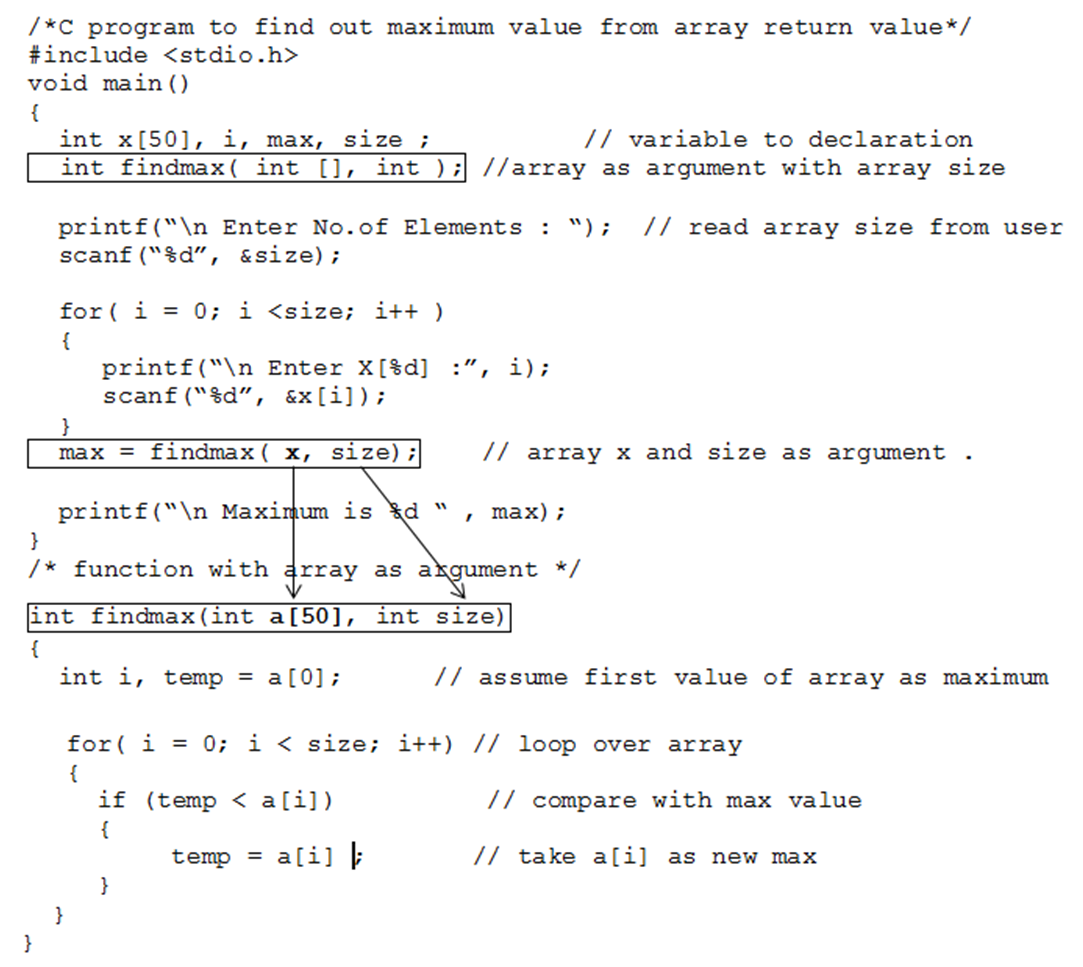• Similar to normal variable, we can also pass an array to the function.
• There is no major difference between passing a variable and array except that we have to specify the dimension for an array so that compiler can differentiate them.
• While passing an array as argument we have to specify array dimension by set of square braces (“[]”) as a function argument at the time of function declaration and function definition.
• There is no difference on calling function with array arguments.
• If array argument is of one dimension, we have to specify single set of square braces (“[]”) and if it is two dimension array, we have to specify two dimension at the time of function declaration and function definition by set of two square braces (“[][]”).
• The following example demonstrates passing array as function argument two find out maximum number from it.Note: By default, Arrays are always passed as pass by reference method

• Here is another example to perform different operations on matrix using array where we have passed 2D-array as function arguments. Please have a look to make it more clear how an array can be used as function arguments.